# 2nd PUC Basic Maths Previous Year Question Paper March 2016

Students can Download 2nd PUC Basic Maths Previous Year Question Paper March 2016, Karnataka 2nd PUC Maths Model Question Papers with Answers helps you to revise the complete Karnataka State Board Syllabus and score more marks in your examinations.

## Karnataka 2nd PUC Basic Maths Previous Year Question Paper March 2016

Time: 3 Hours 15 Min.
Max.Marks: 100

Instructions:

• The question paper has 5 parts A, B, C, D and E. Answer all the pats.
• Part-A carries 10 marks\, Parts – B carries 20 marks, Part – C carries 3 marks, Part – D carries 30 marks and Pat- E carries 1 marks.
• Write the question number properly as indicated in the question paper.

Part – A

Answer all the ten questions: (10 × 1 = 10)

Question 1.
Find x if $$\left[ \begin{matrix} 4 & x \\ x & 16 \end{matrix} \right]$$
$$\left| \begin{matrix} 4 & x \\ x & 16 \end{matrix} \right|$$
64 – x2 ⇒ 0 x2 = 64 ⇒ x = ± 8

Question 2.
How many different arrangements can be made with the letters of the word ‘MONDAY’?
The number of arrangements = 6!

Question 3.
If P and q are propositions with truth values F for false and T for true respectively, find the truth value of ~ q → p
~ q → p is true. ∴ ~(T) → F
F → F = TQuestion 4.
Find the third proportional of 4 and 6.
Let x be the 3rd proportional of 4 and 6,
∴ 4 : 6 :: 6 : x
4x = 36 ⇒ x = 9

Question 5.
What income can be obtained from Rs. 8,000 of 4% stock?
For 100 Rs. → Income 4 Rs.
∴ for 8000 Rs. → $$\frac{8000 \times 4}{100}=320 \mathrm{Rs}$$

Question 6.
IftanA = $$\frac{3}{4}$$ A is acute. Find tan2A.
Given tanA = $$\frac{3}{4}$$Question 7.
If the radius of the circle x2 + y2 + 4x – 2y – k = 0 is 4 units, find k.
g = 2, f = -1,c=-k
$$r=\sqrt{g^{2}+f^{2}-c}$$
$$4=\sqrt{4+1+k} \quad \text { S. } B . S$$
16 = 5 + K ⇒ K = 16 – 5 = 11

Question 8.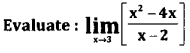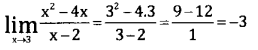Question 9.
If y2 = 4ax find $$\frac{\mathrm{d} y}{\mathrm{d} x}$$
y2 = 4ax
Differentiate with respect to x.
$$\frac{d y}{d x}=\frac{4 a}{2 y}=\frac{2 a}{y}$$

Question 10.
Evaluate : $$\int \frac{3}{x} d x$$
$$\int \frac{3}{x} d x=3 \int \frac{1}{x} d x=3 \log x+c$$

Part – B

Answer any ten questions: (10 × 2 = 20)

Question 11.
If $$\left[ \begin{matrix} 2 & -1 \\ 3 & 1 \end{matrix} \right] \left[ \begin{matrix} x \\ y \end{matrix} \right] =\left[ \begin{matrix} 10 \\ 2 \end{matrix} \right]$$, find x and y.
2x – y = 10
3x + y = 2
Adding we get 5x =12x ⇒ x = $$\frac { 12 }{ 5 }$$ , y = $$2-\frac{3.12}{5}=\frac{10-36}{5}=\frac{-26}{5}$$
∴ x = $$\frac{12}{5}$$ y = $$\frac{-26}{5}$$

Question 12.
If nC8 = nC12 find the value of nC5
nC8 = nC12
nC8 = nCn-12 ⇒ 8 + 12 = n ⇒ n = 20
nC8 = 20C5 = $$\frac{20.19 .18 .17 .16}{5.4 .3 .2 .1}$$ =19 × 3 × 17 × 16 = 15504Question 13.
One ticket is drawn at random from a bag containing 20 tickets numbered 1 to 20. Find the probability that it is a multiple of 2 or 5,
Since the bag has 20 tickets, total number of cases n = 20
Let A: getting a multiple of 2
B: getting a multiple of 5
Event A has 10 favourable outcomes
A = {2, 4, 6, 8, 10, 12, 14, 16, 18, 20}
∴ P(A) = $$\frac{10}{20}=\frac{1}{2}$$
Event B has four favourable outcomes
B = {5, 10, 15, 20)
∴ P(B) = $$\frac{4}{20}$$
A ∩ B = {10,20]
∴ P(A ∩ B) = $$\frac{2}{20}$$
∴ P(multiple of 2 or 5 ) = P(A ∪ B) =P(A) + P(B) – P(A ∩ B) = $$\frac{10}{20}+\frac{4}{20}-\frac{2}{20}=\frac{12}{20}=\frac{3}{5}$$

Question 14.
Write the converse and the contrapositive of the questions are easy then students score better marks.
Given p → q
Converse = q → p If the students score better marks then the questions are easy.
Contrapositive = ~q→ ~p If the student does not score better marks then the questions are not easy.

Question 15.
Monthly incomes of A and B are in the ratio 2:3 and their monthly expenditures are in the ratio 3:5. If each saves Rs. 100 per month, find the monthly incomes of A and B.
Let the monthly incomes be 2x and 3x respectively. Since their savings are Rs. 100 each their expenditure would be 2x – 100 and 3x – 100
∴ $$\frac{2 x-100}{3 x-100}=\frac{3}{5}$$
10x – 500 = 9x – 300; x = Rs. 200
Hence the monthly income of A = 200 × 2 = Rs. 400
The monthly income of B = 200 × 3 = Rs. 600

Question 16.
Find the Banker’s discount on a bill of Rs. 415 due 9 months at 15% p.a
Given F = 415, n = $$\frac{9}{12}$$ , r = 0.15, B.D = ?
B.D = F +r
= 415 × $$\frac{9}{12}$$ × 0.15
B.D = Rs. 46.68Question 17.
Prove that cos3A=4cos3A-3cosA.
L.H.S = cos3A = cos(2A+A)
= cos2A.cosA – sin2A.sinA
= (2cos2A-1)cosA – 2sinA cosA sinA
= 2cos3A – cosA – 2cosA (1-cos2A)
= 2cos3A – cosA – 2cosA +2cos3A = 4 cos3 A – 3 cos A = R.H.S.

Question 18.
Find the value of tna 15°.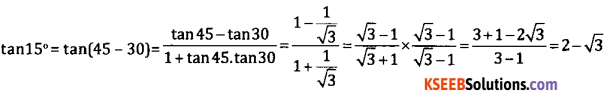Question 19.
Find the equation of the parabola if its vertex is (0,0), axis is y-axis and passes through (-1,-3).
Axis is y-axis and vertex is (0,0).
∴ The parabola is of the form x2 = 4ay and x2 = -4ay. Since the parabola passes through the point (-1,-3) and it lies in third quadrant the parabola turns downward. It is of the form x2 = 4ay but it passes through the point (-1,-3).
∴ (-1)2 = -4a(3).
⇒ 1 = 12a ⇒ a = $$\frac{1}{12}$$
∴ the required equation is x2 = -4. $$\frac{1}{12}$$.y
12x2 + 4y = 0.

Question 20.Given the function is continuous4.1 + 3 = k + 1
7 – 1 = k ⇒ k = 6

Question 21.
If y= log$$\left(\frac{1-\cos x}{1+\cos x}\right)$$ prove that $$\frac{\mathrm{d} y}{\mathrm{d} x}$$ = 2cosecx .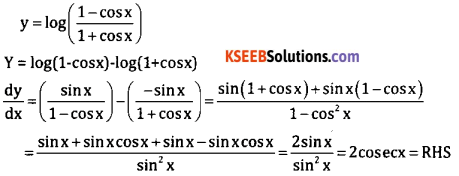Question 22.
If the sum of two numbers is 48, find the numbers whose product is maximum.
Let the two numbers be x and y.
Given x + y = 48
Y = 48 – x
Let product
P = xy = x(48 – x).
P = 48x – x2
Differentiate w.r.t. x.
$$\frac{d p}{d x}$$ = 48 – 2x
For a function to be maximum $$\frac{d p}{d x}$$ = 0
∴ 48 – 2x = 0 ⇒ x = 24 .
∴ y = 48 – 24 = 24 is
∴ product is maximum at x = 24 and y = 24.Question 23.
Evaluate : $$\int \frac{\sin ^{2} x}{1+\cos x} d x$$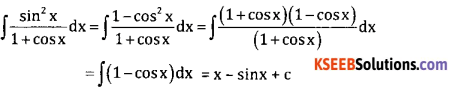Question 24.
Evaluate : $$\int_{\frac{\pi}{4}}^{\frac{\pi}{4}} \csc ^{2} x d x$$
$$\int_{\frac{\pi}{4}}^{\frac{\pi}{4}} \csc 2 x=[-\cot x]_{-\frac{\pi}{4}}^{\frac{\pi}{4}}$$
$$=-\cot \frac{\pi}{4}+\cot \left(\frac{-\pi}{4}\right)=-1-1=-2$$

Part – C

Answer any ten questions: (10 × 3 = 30)

Question 25.
If A = $$\left[ \begin{matrix} 1 & 2 \\ 1 & 4 \end{matrix} \right]$$ B = $$\left[ \begin{matrix} 4 & -3 \\ 2 & 1 \end{matrix} \right]$$ and C = $$\left[ \begin{matrix} 1 & 0 \\ -2 & 4 \end{matrix} \right]$$ show that A(B+C) = AB + AC.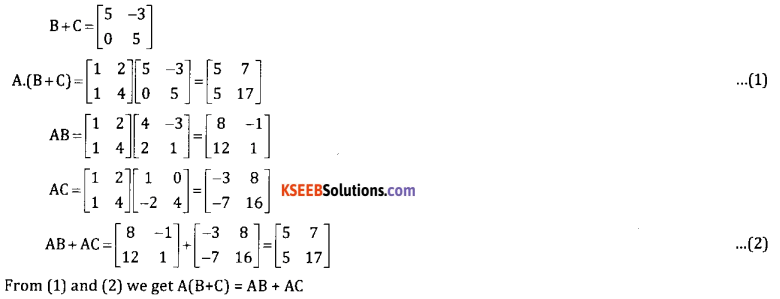Question 26.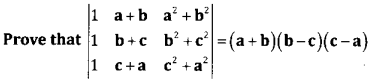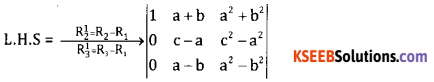Taking c-a and a-b as common factors from Rs and R3(a – b)(c – a).1(a + b – c – a)=(a – b)(b – c)(c – a) = RHS
Appendix B: Examination Question Papers

Question 27.
Find the number of permutations of the letters of the word COMMITTEE. How many of these
(a) Have all the vowels together.
(b) Begin with T and end with T?
Total letters = 9
M = 2, T = 2, E = 2
Total arrangements = $$\frac{9 !}{2 ! 2 ! \cdot 2 !}$$
(a) Permutations begin with T and end with T = $$\frac{7 !}{2 ! 2 !}$$
(b) permutations of vowels are together = $$\frac{6 !}{2 ! \cdot 2 !} \times \frac{4 !}{2 !}$$

Question 28.
A box contains 8 white balls and 9 red balls. Two balls are taken at random from the box. Find the probability that both of them are red if
(i) The two balls are taken together.
(ii) The balls are taken one after the other without replacement.
(iii) The balls are taken one after the other with replacement.
(i) Two balls are selected from 17 balls in 17C2w ways
Ways of choosing 2 Red balls from 9 red is 9C2 ways.
∴ (P both red) = $$\frac{^{9} C_{2}}{^{17} C_{2}}=\frac{9}{34}$$

(ii) Without replacement, probability of 1st red ball = $$\frac{9}{17}$$
And also probability of 2nd red ball is = $$\frac{8}{16}$$

(iii) With replacement
Probability of 1st red ball = $$\frac{9}{17}$$
And also probability of 2nd red ball is = $$\frac{9}{17}$$
∴ P(both red) = $$\frac{9}{17} \times \frac{9}{17}=\frac{81}{289}$$Question 29.
5 carpenters can earn Rs. 540 in 6 days working 9 hours a day. How much will 8 carpenters earn in 12 days
working 6 hours a day?Here all the ratios are direct proportion to 540:x.Hence 8 carpenters can earn Rs. 1920 in 12 days working at the rate of 6 hrs per day.

Question 30.
A banker pays ₹ 2,340 on a ball of 2,500, 146 days before the legally due date. What is the rate of interest charges by the banker?
Given F= 2500, Discounted value = 2340, t = 146 days = $$\frac{146}{365}=\frac{2}{5}$$ r = ?
Discounted value = F(1 – tr)Question 31.
Mr. Sandeep invests ₹15,000 cash partly in 3% stock at 75 and partly in 6% debentures at 125 in such a way as to get a return of 4.5% for his money. How much does he invest in each?
Let x be the investment in 1st stock
And 15,000-x be the investment in 2nd stock
Income in 1st stock = $$\frac{3}{75} \times x$$
Income in 2nd stock = $$\frac{6}{125} \times(15,000-x)$$
Given the sum of both incomes = 675.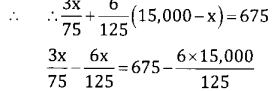(0.04 – 0.048) x = 675 – 720
-0.008 x = – 45
x = 5625
∴ Amount invested in 1st stock = 5625 and
Amount invested 2nd stock = 15,000 – 5625 = Rs. 9375.
Here 4.5% of 15,000 = ₹675

Question 32.
A shopkeeper bought a washing machine at a discount of 20% from a wholesale, the printed price of the washing machine being Rs. 18,000. The shopkeeper sells it to a consumer at a discount of 10% on the printed price. If the rate of sales tax is 8%, find
(i) The VAT paid by the shopkeeper
(ii) Total amount that he consumer pays for the washing machine.
Discount of 10% on 24,000 = 2,400
∴ S.P = 24,000 – 2,400 = 21,600
(i) The amount paid by the cutstomer = 21,600
+ 10% of 21,600 = 21,600 + 2160
The amount paid by the customer = ₹23,760.

(ii) VAT paid by shopkeeper = VAT% (S.P – Shopkeepers S.P)
= 10% (21,600-16,800)Question 33.
Find the focus, equation of directrix and length of lactus rectum of x2 + 16y = 0
x2 = -16y Comparing with
x2 = -4ay we get 4a = 16 ⇒ a = 4
∴ Focus = S = (0,-4)
Directrix is y = -4.
Length of L.R = 4a = 16.

Question 34.
Differentiate xn with respect to x from first principles.
Let y = f(x) = xn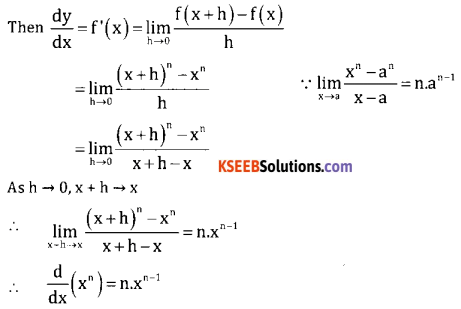Question 35.
The volume of a spherical ball is increasing at the rate of 4π C.C /sec. Find the rate of increase of the radius when the volume is 288π C.C.
Let r be the radius of the sphere and V be the volume of the sphere.
Given $$\frac{\mathrm{d} \mathrm{V}}{\mathrm{dt}}$$ = 4π C.C. /sec., V = 288π C.C.
v = $$\frac{4}{3} \pi r^{3}$$$$\frac{d V}{d t}=4 \pi r^{2} \frac{d r}{d t}$$
$$4 \pi=4 \pi r 2 \frac{d r}{d t} \Rightarrow \frac{d r}{d t}=\frac{1}{r^{2}}=\frac{1}{36} \mathrm{c} \cdot c / \mathrm{sec}$$

Question 36.
Ifs = 2t3 – 5t2 + 4t – 3 (s= distance, t= time), find
(i) The time when the acceleration is 14 ft/sec2.
(ii) The velocity and displacement at that time.
If s = 2t3 – 5t2 + 4t – 3
V = $$\frac{d S}{d t}$$ = 6t2 – 10t + 4
Accn = $$\frac{d V}{d t}$$ =12t – 10
(i) 14 = 12t – 10 ⇒ 14 + 10 = 12t ⇒ t = 2secs.
(ii) Vt=2sec = 6.22 – 10.2 + 4 = 24 – 20 + 4 = 8ft/sec
St = 2sec = 2(2)3 – 5(2)2 + 4.2 + 4 = 16 – 20 + 8 + 4 = 8ft.

Question 37.
Evaluate ∫xsin xdx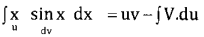= x.(-cosx)- ∫ -cosxdx =-xcosx + sinx+c

Question 38.
Evaluate $$\int \frac{1+e^{x}}{\left(x+e^{x}\right)^{5}} d x$$
$$\int \frac{1+e^{x}}{\left(x+e^{x}\right)^{5}} d x$$
Put x + ex = t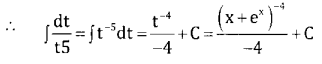Part D

Answer any six questions: (6 × 5 = 30)

Question 39.
Evaluate $$(\sqrt{3}+1)^{5}-(\sqrt{3}-1)^{5}$$ using the binomial theorem.Question 40.
Resolve into partial fractions $$\frac{3 x+5}{(x+2)(x-1)^{2}}$$3x + 5 = A(x – 1)2 + A(x + 2)(x – 1) + C(x + 2)
Put x = -2
-6 + 5 = A(-3)2 ⇒ A = $$\frac { -1 }{ 9 }$$
-1 = A(-3)2 ⇒ A = $$\frac { -1 }{ 9 }$$

Put x = 1,
3 + 5 = A(0) + B(0) + C(1 + 2)
8 = 3C ⇒ C= 7

Put x = 0,
5 = A(-1)2 + B(2)(-1) + C(2)
5 = A – 2B+20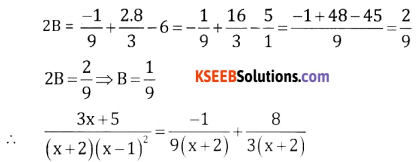Question 41.
Verify whether the proposition [~(p→~q)] v (~p↔q) is a tautology, contradiction or neither.
Let [~(p→~q)] = x and (~p↔q) = y∴ [~(p→~q)] v (~p↔q) is neither tautology nor contradictia

Question 42.
Distribute ₹ 632 amongst A, B and Cin such a way that B will get 20 % more than A and C gets 20% less than B
Express the money received by B and Cas Ratio
i.e. B receives = $$\frac{120}{100}$$ A, if A = 100 then B = 120
$$\therefore \quad \frac{\mathrm{A}}{\mathrm{B}}=\frac{100}{120}=\frac{5}{6}$$
C receives $$\frac{B}{C}=\frac{100}{80}=\frac{5}{4}$$ B. If B = 100, then C = 80
$$\frac{B}{C}=\frac{100}{80}=\frac{5}{4}$$
A : B = 5 : 6; B : C = 5 : 4
A : B : C = 25 : 30 : 24
∴ 25x + 30x + 24x = 632
79x = 632 ⇒ x = $$\frac{632}{79}$$ = 8
∴ A receives 25 × 8 = Rs. 200
B receives 30 × 8 = Rs. 240
C receives 24 × 8 = Rs. 192

Question 43.
XYZ Company supplies water tankers to the government. The first water tanker takes 20,000 labour hours. The government auditors suggest that there should be a 90% learning effect rate. The Management expects an order of 8 water tankers in the next year. What will be the labour cost if the company will incur at the rate of ₹ 20 per hour?
(i) Given 20,000 labour hours = cumulative average
(ii) 90% learning effect
(iii) ₹ 20 per hour
(iv) 8 water tankersTime taken for 8 water tankers =116640 hrs.
Total labour cost = Total no. of hours × per hour 20
116640 × 20 = 2332800Question 44.
Solve the L.P.P. graphically
Maximize z = 6x +8y
Subject to constraints
4x + 2y ≤ 20, 2x + 5y ≤ 24, y ≥ x.
Consider the inequalities as equalities.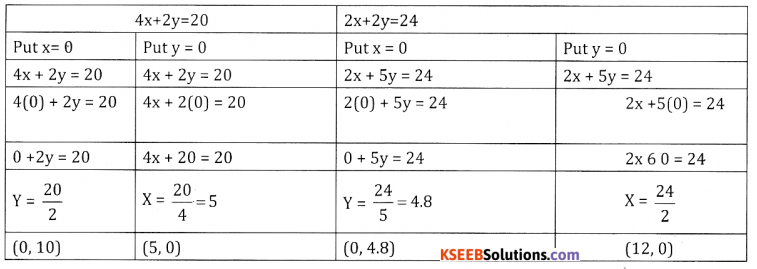4x + 10y = 48
4x + 2y = 20
Sub 8y = 28
y = $$\frac{28}{8}$$ = 3.5
2x = 24 – 5y = 24 – 5y = 24 – 3.5 × 5 = [24 – 17.5]
2x = 6.5
x = 3.25
Point of intersection (3.25, 3.5)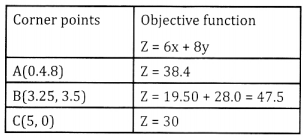Profit is maximum at B(3.25, 3.5) andQuestion 45.
Prove that $$\frac{\cos x+\cos 3 x-\cos 5 x-\cos x}{\sin 7 x-\sin 3 x-\sin 5 x+\sin x}=\cot 2 x$$Question 46.
Find the equation of the circle passing through the point (0,5) and (6, 1) and has its centre on the line 12x + 5y = 25.
Let the equation of the required circle is
x2 + y2 + 2gx + 2fy + c = 0 given that this
Equation passes through the points (0,5) and (6, 1)
∴ (0,5) 02 + 52 + 2(0)g + 2(5)f + C=0
10f + C + 25 = 0
(6, 1) 62 + 12 + 2.6g + 2.1f+C = 0 …. (1)
12g + 2f + C + 37 = 0 …. (2)
Also the centre (-g, -f) lies on the line 12x + 5y – 25 = 0
∴ -128 – 5f – 22 = 0 …. (3)
Equation (2) – Equation (1) gives 12g – 8f + 12 = 0 …(4)
Adding equations 3 and 4 we get
-13f – 13 = 0 → f = $$\frac { 13 }{ -13 }$$ -1 ⇒ f = -1
From equation 4
12g = 8f + 12 = – 8 + 12 = 4
g = $$\frac { 1 }{ 3 }$$
10 + C + 25 = 0 ⇒ c = -15
∴ Required equation is x2 + y2 +2$$\left(\frac{1}{3}\right)$$x + 2(-1)y -15=0
⇒ 3x2 + 3y2 + 2x – y – 45 = 0

Question 47.
If y = acos(logx) + bsin(logx) prove that x2y2+xy1 + y = 0
If y = acos(logx) + bsin(logx)
$$y_{1}=\frac{-a \sin (\log x)}{x}+\frac{b \cos (\log x)}{x}$$
xy1 = -asin(logx) + bcos(logy)
again diff. w.rit. x.
$$\mathrm{xy}_{2}+\mathrm{y}_{1} \cdot 1=\frac{-\mathrm{a} \cos (\log \mathrm{x})}{\mathrm{x}}-\frac{\mathrm{b} \sin (\log \mathrm{x})}{\mathrm{x}}$$
x2y2 + xy1 = – y ⇒ x2y2 + xy1 + y = 0Question 48.
Find the area bounded by the parabola y2 = 5x and the line y = x.
y2 = 5x and y = x
⇒ x2 = 5
⇒ x2 – 5x = 0
⇒ x(x – 5) = 0 ⇒ x = 0 or x = 5 are the limits
Required area A = |A1 – A2|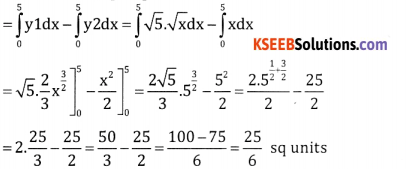Part – E

Answer any one question: (1 × 10 = 10)

Question 49.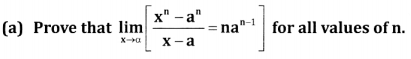Case 1: Let n be a positive integer.
xn – an = (x-a)(xn-1 + xn-2. a + xn-3. a2 + ———- + 2n-1)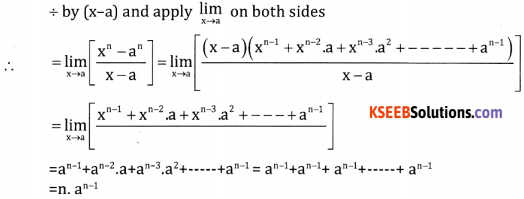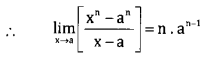Case 2: Let n be a negative integer
put n = -m, m > 0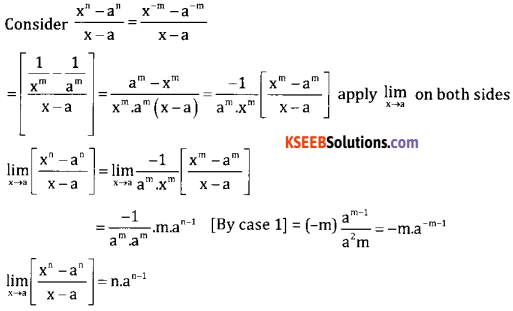Case 3: Let n = p/q where p and q are integers and q ≠ 0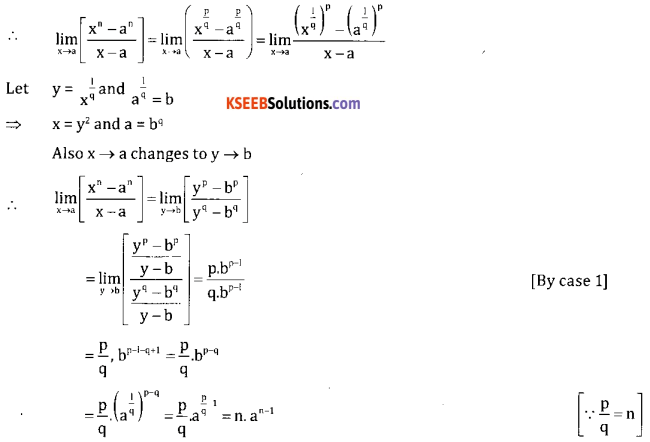Hence it proved for al the values of n

(b) Expand (0.99) by Binomial theorem correctly four decimal places.
(0.99)5 = (1 – 0.01)5 = 155 + 5C1 . 14.(-0.01)+5C2.13(-0.01)2 + 5C3.12(-0.01)4 + 5C4(-0.01)5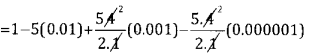= 1 – 0.05 + 0.0010 – 0.00001 = 1.001 – 0.05001 = 1.95099 ∴ (0.99)5 = 1.95099Question 50.
(a) Transport corporation operates bus service between two villages. Data regarding the passenger traffic during the first three days of the week is given below along with the total 4 revenue.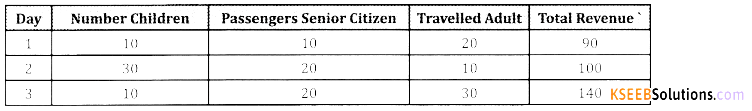Find the bus far charged per children, per senior citizen and per adult by using matrix method.
Let x, y & z denote the rates of Commission in Rupees/ unit for A,B&C iterms respectively. Then the data given can be expressed as a system of linear equations.
10x + 10y + 20z =90
30x + 20y + 10z = 100
10x + 20y + 30z = 140
x + y + 2x = 9
3x + 2y + z = 10
x + 2y + 3z = 14
Solve these equations by Matrix Method.b. Two towers of height 14m and 25cm stand on level ground. The angles of elevation of their tops from a point on the line joining their feet are 450 and 600 respectively. Find the distance between the towers.
Let AB = 25m, DE = 14m are two towers.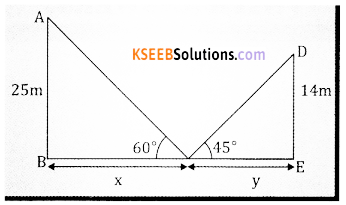Let BC = x and CE = y, x + y = ?
From rt. Angled triangle ∆ce DCE
tan 60o = $$\frac{20}{x} \Rightarrow \sqrt{3}=\frac{25}{x} \Rightarrow x=\frac{25}{\sqrt{3}}$$ … (1)
Again form right angled ∆Ce DCE
tan 45o = $$\frac{14}{y}$$ ⇒ y = 14 …. (2)
∴ tan 45 = 1
From Equations (1) and (2) we get.
$$x+y \frac{25}{\sqrt{3}}+14=\frac{25+14 \sqrt{3}}{\sqrt{3}}$$
Hence the distance between the towers = $$\frac{25+4 \sqrt{3}}{\sqrt{3}}$$ mt3

error: Content is protected !!